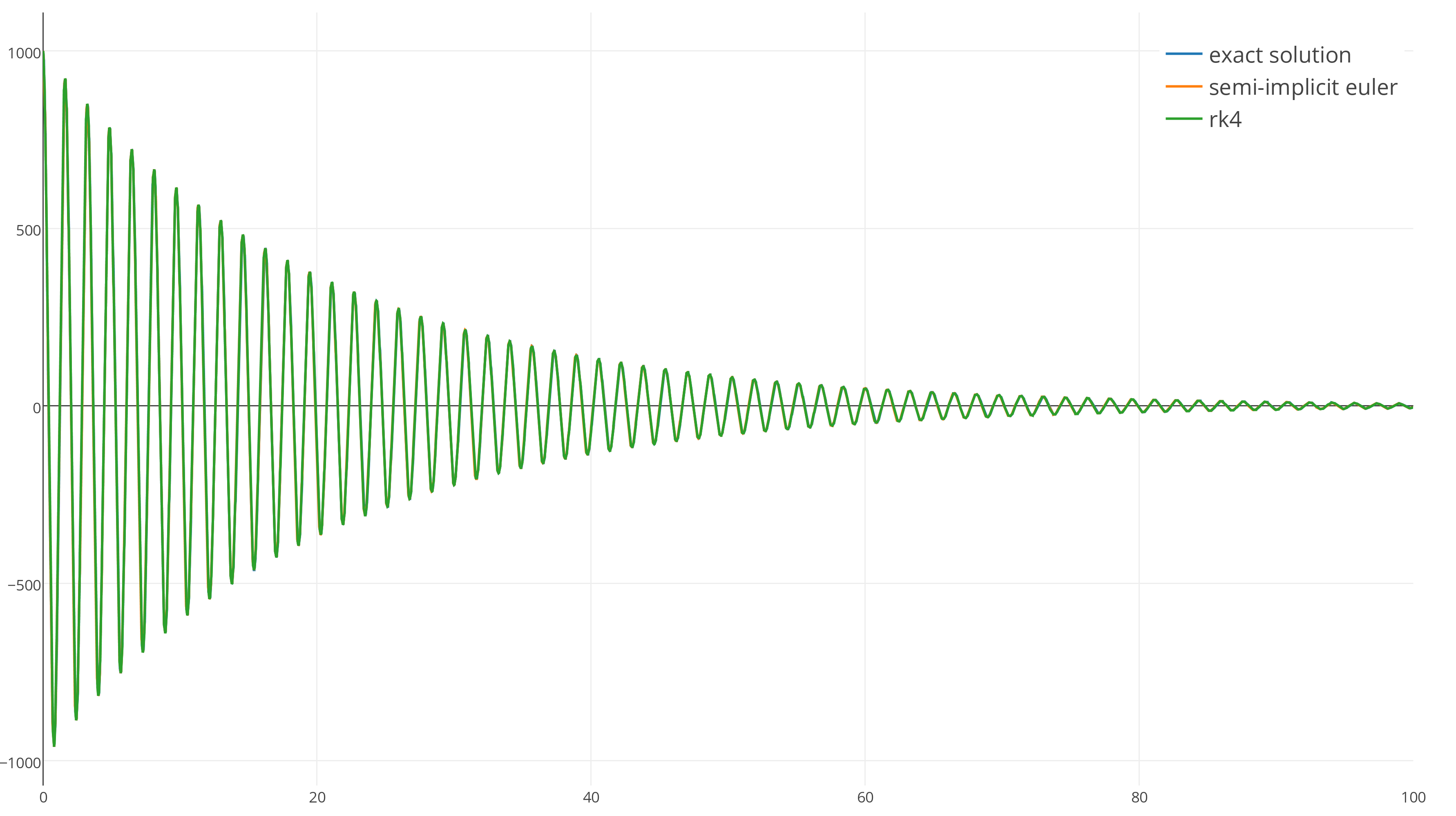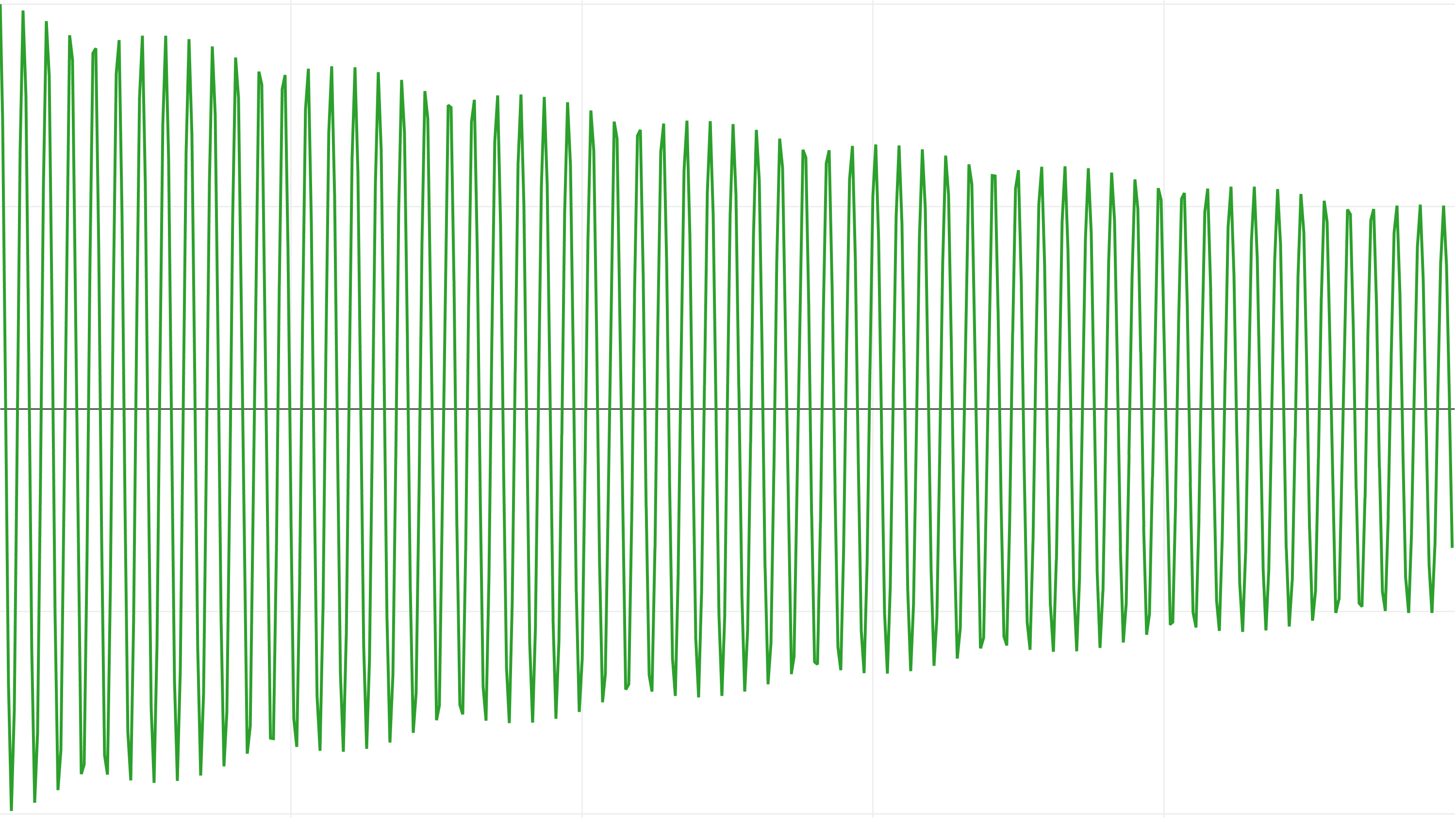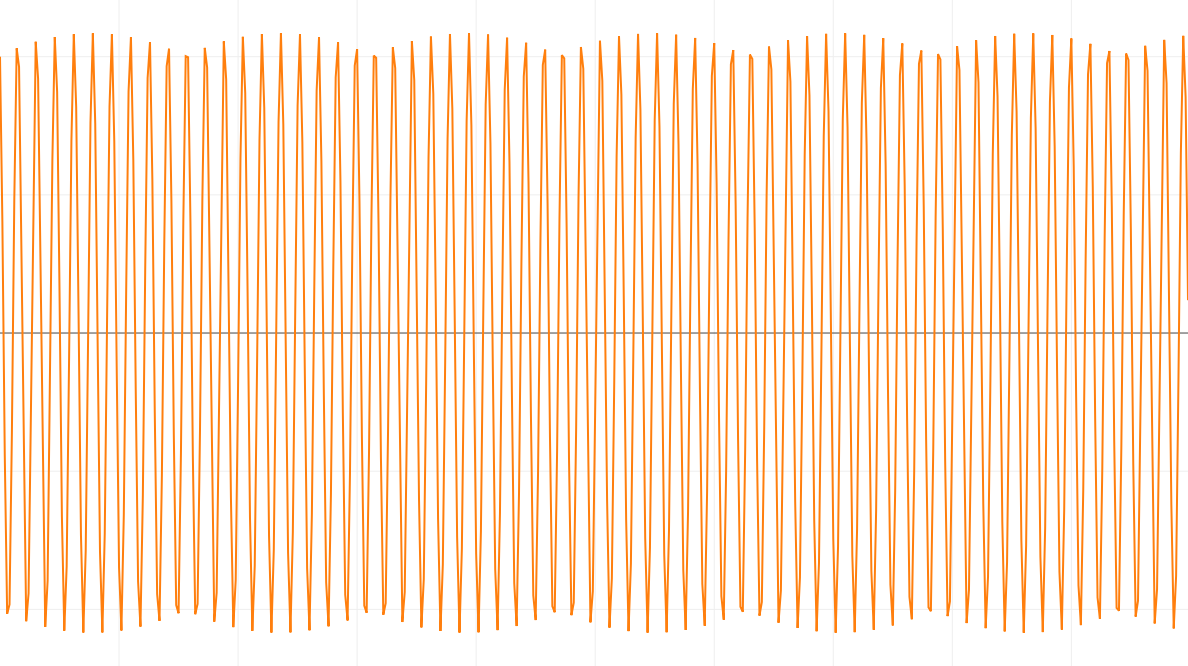# Integration Basics

## Introduction

Hi, I’m Glenn Fiedler and welcome to Game Physics.

If you have ever wondered how the physics simulation in a computer game works then this series of articles will explain it for you. I assume you are proficient with C++ and have a basic grasp of physics and mathematics. Nothing else will be required if you pay attention and study the example source code.

A physics simulation works by making many small predictions based on the laws of physics. These predictions are actually quite simple, and basically boil down to something like “the object is here, and is traveling this fast in that direction, so in a short amount of time it should be over there”. We perform these predictions using a mathematical technique called integration.

Exactly how to implement this integration is the subject of this article.

## Integrating the Equations of Motion

You may remember from high school or university physics that force equals mass times acceleration.

    F = ma


We can switch this around to see that acceleration is force divided by mass. This makes intuitive sense because heavier objects are harder to throw.

    a = F/m


Acceleration is the rate of change in velocity over time:

    dv/dt = a = F/m


Similarly, velocity is the rate of change in position over time:

    dx/dt = v


This means if we know the current position and velocity of an object, and the forces that will be applied to it, we can integrate to find its position and velocity at some point in the future.

## Numerical Integration

For those who have not formally studied differential equations at university, take heart for you are in almost as good a position as those who have. This is because we’re not going to analytically solve the differential equations as you would do in first year mathematics. Instead, we are going to numerically integrate to find the solution.

Here is how numerical integration works. First, start at an initial position and velocity, then take a small step forward to find the velocity and position at a future time. Then repeat this, moving forward in small time steps, using the result of the previous calculation as the starting point for the next.

But how do we find the change in velocity and position at each step?

The answer lies in the equations of motion.

Let’s call our current time t, and the time step dt or ‘delta time’.

We can now put the equations of motion in a form that anyone can understand:

    acceleration = force / mass
change in position = velocity * dt
change in velocity = acceleration * dt


This makes intuitive sense because if you’re in a car traveling 60 kilometers per-hour, in one hour you’ll be 60 kilometers further down the road. Similarly, a car accelerating 10 kilometers per-hour-per-second will be moving 100 kilometers per-hour faster after 10 seconds.

Of course this logic only holds when acceleration and velocity are constant. But even when they’re not, it’s still a pretty decent approximation to start with.

Let’s put this into code. Starting with a stationary object at the origin weighing one kilogram, we apply a constant force of 10 newtons and step forward with time steps of one second:

    double t = 0.0;
float dt = 1.0f;

float velocity = 0.0f;
float position = 0.0f;
float force = 10.0f;
float mass = 1.0f;

while ( t <= 10.0 )
{
position = position + velocity * dt;
velocity = velocity + ( force / mass ) * dt;
t += dt;
}


Here is the result:

    t=0:    position = 0      velocity = 0
t=1:    position = 0      velocity = 10
t=2:    position = 10     velocity = 20
t=3:    position = 30     velocity = 30
t=4:    position = 60     velocity = 40
t=5:    position = 100    velocity = 50
t=6:    position = 150    velocity = 60
t=7:    position = 210    velocity = 70
t=8:    position = 280    velocity = 80
t=9:    position = 360    velocity = 90
t=10:   position = 450    velocity = 100


As you can see, at at each step we know both the position and velocity of the object. This is numerical integration.

## Explicit Euler

What we just did is a type of integration called explicit euler.

To save you future embarrassment, I must point out now that Euler is pronounced “Oiler” not “yew-ler” as it is the last name of the Swiss mathematician Leonhard Euler who first discovered this technique.

Euler integration is the most basic numerical integration technique. It is only 100% accurate when the rate of change is constant over the timestep.

Since acceleration is constant in the example above, the integration of velocity is without error. However, we are also integrating velocity to get position, and velocity is increasing due to acceleration. This means there is error in the integrated position.

Just how large is this error? Let’s find out!

There is a closed form solution for how an object moves under constant acceleration. We can use this to compare our numerically integrated position with the exact result:

    s = ut + 0.5at^2
s = 0.0*t + 0.5at^2
s = 0.5(10)(10^2)
s = 0.5(10)(100)
s = 500 meters


After 10 seconds, the object should have moved 500 meters, but explicit euler gives a result of 450 meters. That’s 50 meters off after just 10 seconds!

This sounds really, really bad, but it’s not common for games to step physics forward with such large time steps. In fact, physics usually steps forward at something closer to the display framerate.

Stepping forward with dt = 1/100 yields a much better result:

    t=9.90:     position = 489.552155     velocity = 98.999062
t=9.91:     position = 490.542145     velocity = 99.099060
t=9.92:     position = 491.533142     velocity = 99.199059
t=9.93:     position = 492.525146     velocity = 99.299057
t=9.94:     position = 493.518127     velocity = 99.399055
t=9.95:     position = 494.512115     velocity = 99.499054
t=9.96:     position = 495.507111     velocity = 99.599052
t=9.97:     position = 496.503113     velocity = 99.699051
t=9.98:     position = 497.500092     velocity = 99.799049
t=9.99:     position = 498.498077     velocity = 99.899048
t=10.00:    position = 499.497070     velocity = 99.999046


As you can see, this is a pretty good result. Certainly good enough for a game.

## Why explicit euler is not (always) so great

With a small enough timestep explicit euler gives decent results for constant acceleration, but what about the case where acceleration isn’t constant?

A good example of non-constant acceleration is a spring damper system.

In this system a mass is attached to a spring and its motion is damped by some kind of friction. There is a force proportional to the distance of the object that pulls it towards the origin, and a force proportional to the velocity of the object, but in the opposite direction, which slows it down.

Now the acceleration is definitely not constant throughout the timestep, but is a continously changing function that is a combination of the position and velocity, which are themselves changing continuously over the timestep.

This is an example of a damped harmonic oscillator. It’s a well studied problem and there’s a closed form solution that we can use to check our numerically integrated result.

Here are the input parameters to the mass spring system:

• Mass: 1 kilogram
• Initial position: 1000 meters from origin
• Hooke’s law spring coefficient: k = 15
• Hooke’s law damping coefficient: b = 0.1

And here is a graph of the exact solution:When we apply explicit euler to integrate this system, we get the following result, which has been scaled down vertically to fit:Instead of damping and converging on the origin, it gains energy over time!

This system is unstable when integrated with explicit euler and dt=1/100.

Unfortunately, since we’re already integrating with a small timestep, we don’t have a lot of practical options to improve the accuracy. Even if you reduce the timestep, there’s always a spring tightness k above which you’ll see this behavior.

## Semi-implicit Euler

Another integrator to consider is semi-implicit euler.

Most commercial game physics engines use this integrator.

Switching from explicit to semi-implicit euler is as simple as changing:

    position += velocity * dt;
velocity += acceleration * dt;


to:

    velocity += acceleration * dt;
position += velocity * dt;


Applying the semi-implicit euler integrator with dt = 1/100 to the spring damper system gives a stable result that is very close to the exact solution:Even though semi-implicit euler has the same order of accuracy as explicit euler (order 1), we get a much better result when integrating the equations of motion because it is symplectic.

## Many different integration methods exist

Implicit euler is an integration technique that is well suited for simulating stiff equations that become unstable with other methods. The drawback is that it requires solving a system of equations per-timestep.

Verlet integration provides greater accuracy than implicit euler and less memory usage when simulating a large number of particles is. This is a second order integrator which is also symplectic.

There are a whole family of integrators called the Runge-Kutta methods. Explicit euler is part of this family, but it also includes higher order integrators, the most classic of these being the Runge Kutta order 4 or simply RK4.

This Runge Kutta family of integrators is named for the German physicists who discovered them: Carl Runge and Martin Kutta. This means the ‘g’ is hard and the ‘u’ is a short ‘oo’ sound. I am sorry to inform but this means we are talking about the ‘roon-geh koo-ta’ methods and not a ‘runge cutter’, whatever that is :)

The RK4 is a fourth order integrator, which means its accumulated error is on the order of the fourth derivative. This makes it very accurate. Much more accurate than explicit and implicit euler which are only first order.

But although it’s more accurate, that’s not to say RK4 is automatically “the best” integrator, or that it is better than semi-implicit euler. It’s much more complicated than this.

Regardless, it’s an interesting integrator and is well worth studying.

## Implementing RK4

There are many great explanations of the mathematics behind RK4 already. For example: here, here and here. I highly encourage you to follow the derivation and understand how and why it works at a mathematical level. But, seeing as the target audience for this article are programmers, not mathematicians, we’re all about implementation, so let’s get started.

Let’s define the state of an object as a struct in C++ so we have both position and velocity stored conveniently in one place:

    struct State
{
float x;      // position
float v;      // velocity
};


We also need a struct to store the derivatives of the state values:

    struct Derivative
{
float dx;      // dx/dt = velocity
float dv;      // dv/dt = acceleration
};


Next we need a function to advance the physics state ahead from t to t+dt using one set of derivatives, and once there, recalculate the derivatives at this new state:

    Derivative evaluate( const State & initial,
double t,
float dt,
const Derivative & d )
{
State state;
state.x = initial.x + d.dx*dt;
state.v = initial.v + d.dv*dt;

Derivative output;
output.dx = state.v;
output.dv = acceleration( state, t+dt );
return output;
}


The acceleration function is what drives the entire simulation. Let’s set it to the spring damper system and return the acceleration assuming unit mass:

    float acceleration( const State & state, double t )
{
const float k = 15.0f;
const float b = 0.1f;
return -k * state.x - b * state.v;
}


Now we get to the RK4 integration routine itself:

    void integrate( State & state,
double t,
float dt )
{
Derivative a,b,c,d;

a = evaluate( state, t, 0.0f, Derivative() );
b = evaluate( state, t, dt*0.5f, a );
c = evaluate( state, t, dt*0.5f, b );
d = evaluate( state, t, dt, c );

float dxdt = 1.0f / 6.0f *
( a.dx + 2.0f * ( b.dx + c.dx ) + d.dx );

float dvdt = 1.0f / 6.0f *
( a.dv + 2.0f * ( b.dv + c.dv ) + d.dv );

state.x = state.x + dxdt * dt;
state.v = state.v + dvdt * dt;
}


The RK4 integrator samples the derivative at four points to detect curvative. Notice how derivative a is used when calculating b, b is used when calculating c, and c into d. This feedback of the current derivative into the calculation of the next is what gives the RK4 integrator its accuracy.

Importantly, each of these derivatives a,b,c and d will be different when the rate of change in these quantities is a function of time or a function of the state itself. For example, in our spring damper system acceleration is a function of the current position and velocity which change throughout the timestep.

Once the four derivatives have been evaluated, the best overall derivative is calculated as a weighted sum derived from the taylor series expansion. This combined derivative is used to advance the position and velocity forward, just as we did with the explicit euler integrator.

## Semi-implicit euler vs. RK4

Now let’s put the RK4 integrator to the test.

Since it is a higher order integrator (4th order vs. 1st order) it will be visibly more accurate than semi-implicit euler, right?Wrong. Both integrators are so close to the exact result that it’s impossible to make out any difference at this scale. Both integrators are stable and track the exact solution very well with dt=1/100.Zooming in confirms that RK4 is more accurate than semi-implicit euler, but is it really worth the complexity and extra runtime cost of RK4? It’s hard to say.

Let’s push a bit harder and see if we can find a significant difference between the two integrators. Unfortunately, we can’t look at this system for long periods of time because it quickly damps down to zero, so let’s switch to a simple harmonic oscillator which oscillates forever without any damping.

Here’s the exact result we’re aiming for:To make it harder on the integrators, let’s increase delta time to 0.1 seconds.

Next, we let the integrators run for 90 seconds and zoom in:After 90 seconds the semi-implicit euler solution (orange) has drifted out of phase with the exact solution because it has a slightly different frequency, while the green line of RK4 matches the frequency, but is losing energy!

We can see this more clearly by increasing the time step to 0.25 seconds.

RK4 maintains the correct frequency but loses energy:While semi-implicit euler does a better job at conserving energy, on average:But drifts out of phase. What an interesting result! As you can see it’s not simply the case that RK4 has a higher order of accuracy and is “better”. It’s much, much more nuanced than this.

## Conclusion

Which integrator should you use in your game?

My recommendation is semi-implicit euler. It’s cheap and easy to implement, it’s much more stable than explicit euler, and it tends to preserve energy on average even when pushed near its limit.

If you really do need more accuracy than semi-implicit euler, I recommend you look into higher order symplectic integrators designed for hamiltonian systems. This way you’ll discover more modern higher order integration techniques that are better suited to your simulation than RK4.

And finally, if you are still doing this in your game:

    position += velocity * dt;
velocity += acceleration * dt;


Please take a moment to change it to this:

    velocity += acceleration * dt;
position += velocity * dt;


You’ll be glad you did :)